# Holomorphically-convex complex space

(diff) ← Older revision | Latest revision (diff) | Newer revision → (diff)

A complex spacethat satisfies the following condition: For each compactum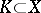the set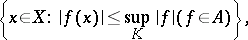whereis the algebra of holomorphic functions on, is compact. A spaceis holomorphically convex if and only if it admits a proper surjective holomorphic mappingonto some Stein space (a holomorphically-complete space)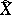which induces an isomorphism between the algebras of holomorphic functions on these spaces. The mapping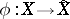(the holomorphic reduction of) is uniquely defined and has connected fibres . For any coherent analytic sheafon a holomorphically-convex complex space, the cohomology spaces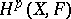and,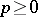, are separable topological vector spaces .

A special class of holomorphically-convex complex spaces is formed by the complex spacesfor which the holomorphic reduction mapping is bijective outside some compact analytic set (such a space is obtained from a Stein space by a proper modification which blows-up a finite number of points). A complex space possesses this property if and only if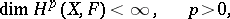for any coherent analytic sheafon. This class of complex spaces also coincides with the class of strictly-convex complex spaces (cf. Pseudo-convex and pseudo-concave).

How to Cite This Entry:
Holomorphically-convex complex space. Encyclopedia of Mathematics. URL: http://encyclopediaofmath.org/index.php?title=Holomorphically-convex_complex_space&oldid=16349
This article was adapted from an original article by A.L. Onishchik (originator), which appeared in Encyclopedia of Mathematics - ISBN 1402006098. See original article